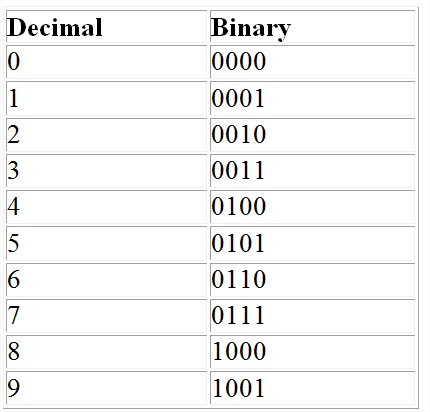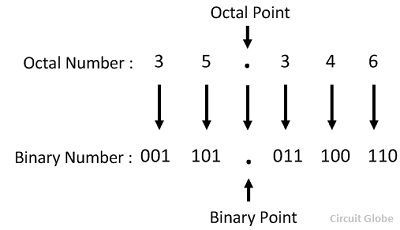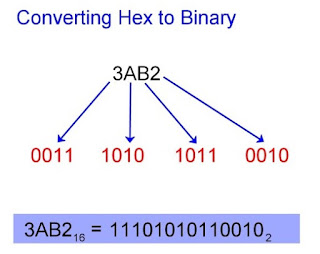### Common Base ConversionsSource: https://www.tes.com/teaching-resource/0-9-digit-cards-6044974
When we represent numbers in mathematics, we utilize ten digits zero to nine to represent all numbers. In computer science, this is called the base 10 system because it has ten digits. There are three other common bases that are studied in C.S.: base 2, 8 and 16, with two, eight and sixteen digits, respectively. Before we examine each of these in more detail, your are probably asking...

Why do we need to learn manual base conversions?

Although humans read in base-10, computers read in base-2 as the internal mechanisms can only reliably provide two options (i.e. are switches) that make data storage only possible in binary. But as the number gets larger the characters of binary get much larger and unwieldy to work with when examining computer codes. In the past, base-8 was used to shorten these long strings of numbers into more manageable chunks, but today this base-16 has supplanted octal in this role because it is more efficient. Now, we will move onto how to convert between these systems. We will use "binary" as a base case from which you can access the other bases (i.e. you would not convert directly from decimal to octal, but from decimal to binary and then from binary to octal).

Binary and Decimal:

First we examine the question of how to convert between our native number system and binary.Source: https://www.geeksforgeeks.org/program-binary-decimal-conversion/
It is pretty intuitive to convert from binary to decimal. This is a base two system, and each extra digit represents a larger power of two. Since we only have two options, you will add either that power of two or not depending on whether the digit is zero or one.Source: https://brilliant.org/wiki/binary-coded-decimal-or-bcd/
This table tells you how the decimal digits themselves are converted to binary numbers and vice-versa.Source: https://owlcation.com/stem/How-to-Convert-Decimal-to-Binary-and-Binary-to-Decimal
There is also a rather intuitive way to convert decimals to binary using long division as shown above. This also relies on the powers of two, but instead of multiplication as in the prior conversion it uses division.

Binary and Octal:Source: https://www.wikihow.com/Convert-Binary-to-Octal-Number
The relationship between octal and binary is based on this 4-2-1 relationship based on the cluster of the binary number, starting from the right in groups of three. If the final group (the most left-most or at the front of the number), does not have three, then you add on zeroes until you reach three. Then you write 4-2-1 and multiply by the available digits to generate the octal digits.Another example of binary to octal: https://circuitglobe.com/octal-to-binary-and-binary-to-octal-conversion-methods.htmlChart for the octal digits: https://www.youtube.com/watch?v=a4i1OnDtufc
Converting from octal to binary requires an intuitive sense of the top chart (or the 4-2-1 rule). You convert each octal digit back into its component three binary digits to get your final value.Octal to Binary: https://circuitglobe.com/octal-to-binary-and-binary-to-octal-conversion-methods.htmlSource: https://owlcation.com/stem/How-to-Convert-Hex-to-Binary-and-Binary-to-Hexadecimal
Hexadecimal relies on the very same principle as octal except with groups of four binary digits instead of three (thereby creating an 8-4-2-1 rule). The system adds extra digits A (10), B (11), C (12), D (13), E (14), F (15). I've added the decimal equivalents because it makes it easier to understand what they represent. This four-digit system allows for manual conversion forwards and backwards as with the previous system.

Extra Sources
1.